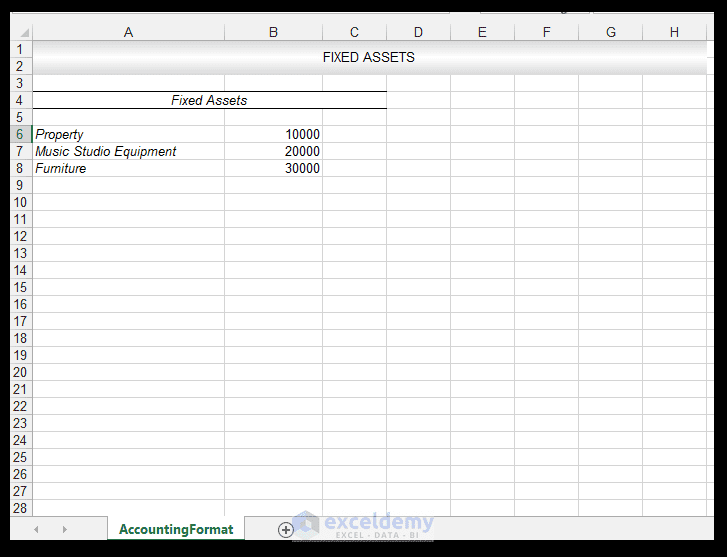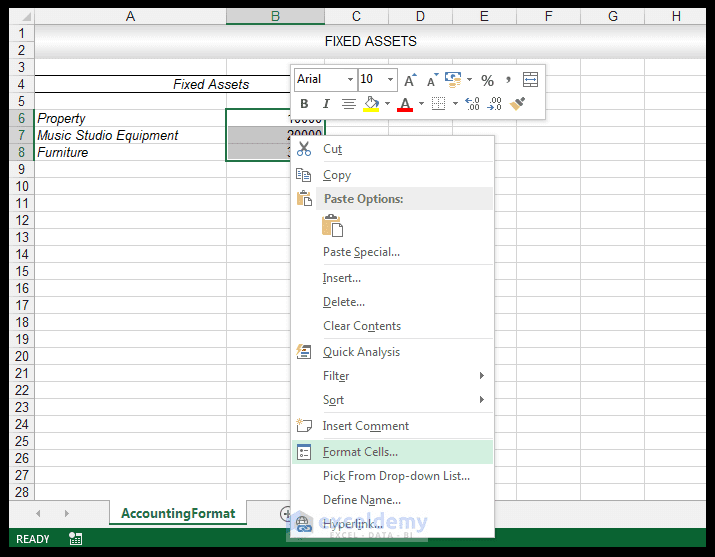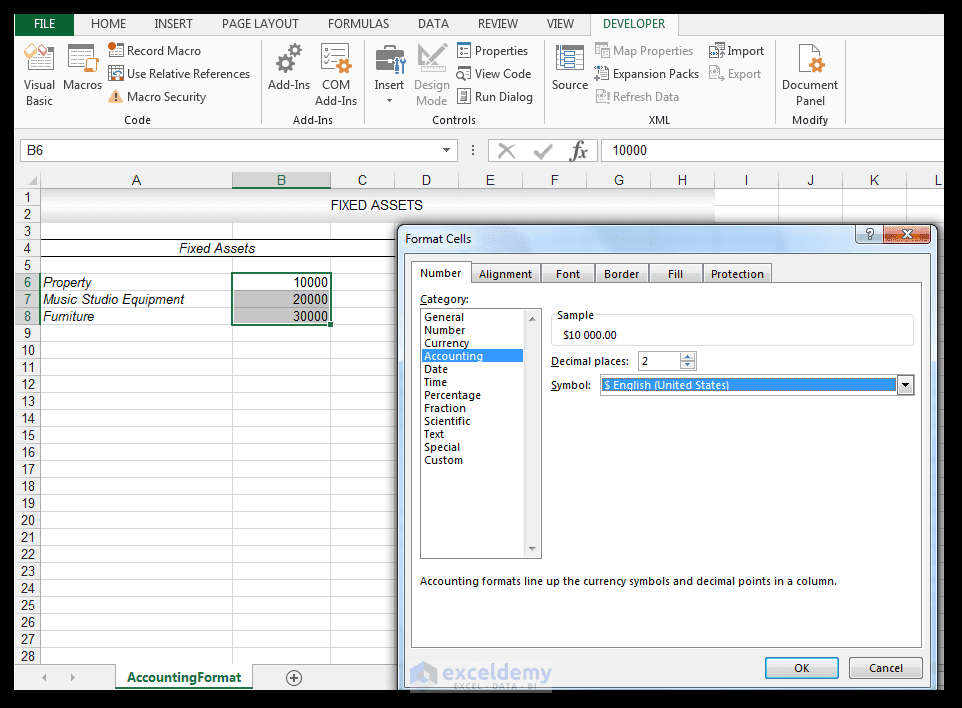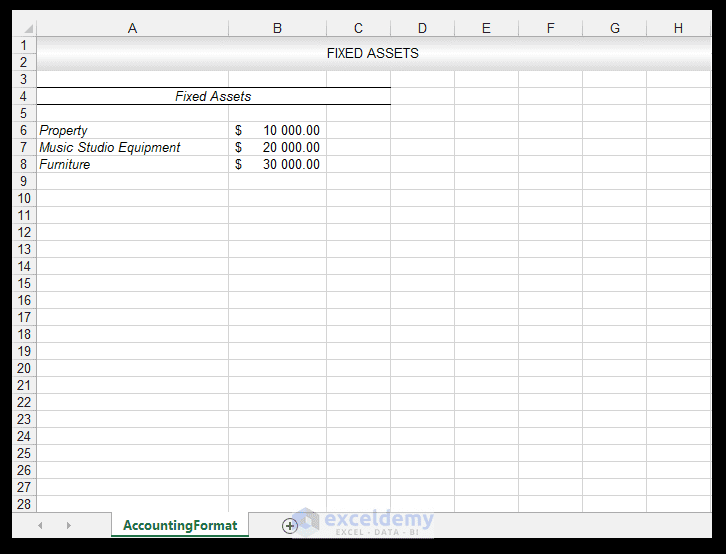How to Apply Accounting Number Format in Excel!

The Accounting Format is similar to the Currency format and can be applied to numbers where needed. The difference between the Accounting format and the Currency format is that the Accounting format puts the dollar sign for example, at the far left end of the cell, and displays zero as a dash. So, let’s look at a simple example in order to see how to apply Accounting number format in Excel.

How to Apply Accounting Number Format in Excel

An accountant working at a hypothetical accountancy firm is compiling a spreadsheet noting the value of fixed assets, for the current financial year as shown below.1) In order to apply Accounting format, select the range and right-click and choose Format Cells.2) In the Format Cells Dialog box, with the Number Tab selected, choose Accounting and accept the options shown below and click Ok.3) The Accounting Format is thus applied.And there you have it.

ApplyingAccountingFormat

Conclusion

Applying the Accounting format is simple and straightforward in Excel. One can use the Accounting format when compiling financial statements, balance sheets and for other Accountancy related purposes.